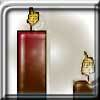#### You may also like### Burning Down

One night two candles were lit. Can you work out how long each candle was originally?### Percentage Unchanged

If the base of a rectangle is increased by 10% and the area is unchanged, by what percentage is the width decreased by ?### Digit Sum

What is the sum of all the digits in all the integers from one to one million?

# Cancelling Fractions

##### Age 14 to 16 ShortChallenge Level

Using factorials
Choose small numerators, say $2, 3, 4, 5, 6, 7$
$2\times3\times4\times5\times6 \times 7= m$ would give $(m+2)$ divisible by $2$,
$(m+2) + 1$ divisible by $3$,
$(m+2) + 2$ divisible by $4$,
.....
$(m+2) + 5$ divisible by $7$

So $n=2$, and $m=2\times3\times4\times5\times6\times7+2$

Using smaller numbers
From the method above, we could have started from any number that was a multiple of $7$, $6$, $5,$ $4,$ $3$ and $2$ - so we could have started from the lowest common multiple of $7$, $6$, $5,$ $4,$ $3$ and $2,$ which is $7\times5\times4\times3= 420$. So, if $n=420+2=422$ and $m=2,$ the cancelling fractions are $$\frac{2}{422},\frac{3}{423},\frac{4}{424},\frac{5}{425},\frac{6}{426},\frac{7}{427}$$

Starting from $m=2$
Look for an $n$ corresponding to $m=2$. Then we require $n$ to have factor $2$, $n+1$ to have factor $3$ and so on. Hence $n$ cannot have any of the factors $3, 4, 5, 6$ or $7$. Since $n+3$ must be divisible by $5$, we must have $n$ ending in a $2$ or a $7$, but $n$ is even so it must end in a $2$.

In order for $n+2$ to be divisible by $4$, the number must be made up of an even number of tens with remainder 2. Since $n+1$ is divisible by $3$, we must also have the number of tens divisible by $3$.

The final condition is that our number which we have now determined to be of the form $n=60k+2$ is $5$ less than a multiple of $7$ ($n+4$ is automatically divisible by $6$). This is clearly true for $k = 7$, so $n = 422$ gives the fraction $$\frac{2}{422}$$ with the required property.

You can find more short problems, arranged by curriculum topic, in our short problems collection.